Test: Alligation or Mixture- 2

# Test: Alligation or Mixture- 2

Test Description

## 10 Questions MCQ Test CSAT Preparation for UPSC CSE | Test: Alligation or Mixture- 2

Test: Alligation or Mixture- 2 for Banking Exams 2023 is part of CSAT Preparation for UPSC CSE preparation. The Test: Alligation or Mixture- 2 questions and answers have been prepared according to the Banking Exams exam syllabus.The Test: Alligation or Mixture- 2 MCQs are made for Banking Exams 2023 Exam. Find important definitions, questions, notes, meanings, examples, exercises, MCQs and online tests for Test: Alligation or Mixture- 2 below.
Solutions of Test: Alligation or Mixture- 2 questions in English are available as part of our CSAT Preparation for UPSC CSE for Banking Exams & Test: Alligation or Mixture- 2 solutions in Hindi for CSAT Preparation for UPSC CSE course. Download more important topics, notes, lectures and mock test series for Banking Exams Exam by signing up for free. Attempt Test: Alligation or Mixture- 2 | 10 questions in 20 minutes | Mock test for Banking Exams preparation | Free important questions MCQ to study CSAT Preparation for UPSC CSE for Banking Exams Exam | Download free PDF with solutions
 1 Crore+ students have signed up on EduRev. Have you?
Test: Alligation or Mixture- 2 - Question 1

### In what ratio must rice at Rs.7.10 be mixed with rice at Rs.9.20 so that the mixture may be worth Rs.8 per Kg?

Test: Alligation or Mixture- 2 - Question 2

### In what ratio must tea at Rs.62 per Kg be mixed with tea at Rs. 72 per Kg so that the mixture must be worth Rs. 64.50 per Kg?

Test: Alligation or Mixture- 2 - Question 3

### A vessel is filled with liquid, 3 parts of which are water and 5 parts of syrup. How much of the mixture must be drawn off and replaced with water so that the mixture may be half water and half syrup?

Detailed Solution for Test: Alligation or Mixture- 2 - Question 3

Let initially vessel have 8 l of liquid and x lof this liquid be replaced with water then quantity of water in new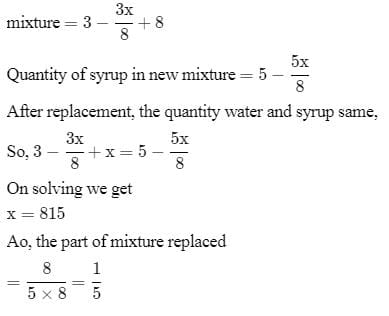Test: Alligation or Mixture- 2 - Question 4

A container contains a mixture of two liquids P and Q in the ratio 7 : 5. When 9 litres of mixture are drawn off and the container is filled with Q, the ratio of P and Q becomes 7 : 9. How many litres of liquid P was contained in the container initially?

Detailed Solution for Test: Alligation or Mixture- 2 - Question 4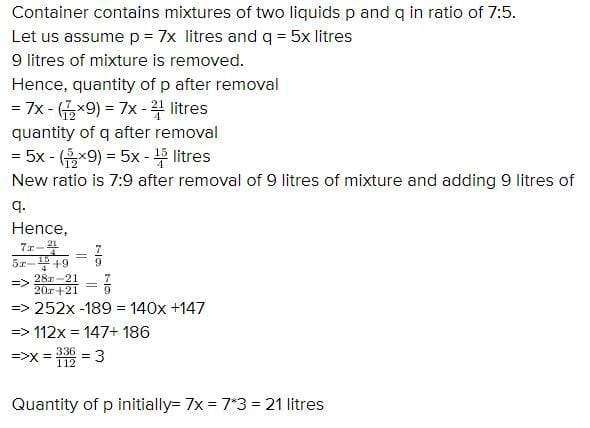Test: Alligation or Mixture- 2 - Question 5

In what ratio must a grocer mix two varieties of pulses costing Rs.15 and Rs. 20 per kg respectively to obtain a mixture worth Rs.16.50 per Kg?

Detailed Solution for Test: Alligation or Mixture- 2 - Question 5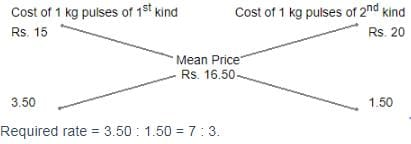Test: Alligation or Mixture- 2 - Question 6

In what ratio must tea worth Rs. 60 per kg be mixed with tea worth Rs. 65 a Kg such that by selling the mixture at Rs. 68.20 a Kg ,there can be a gain 10%?

Detailed Solution for Test: Alligation or Mixture- 2 - Question 6

100/110 × 68.20 = 62
Let the amount of tea worth rs 60 be x and the amount of tea worth rs 65 be y
The total amount is : x + y
60x + 65y = 62(x + y)
60x + 65y = 62x + 62y
65y - 62y = 62x - 60x
3y = 2x
3/2 = x / y
3 : 2

Test: Alligation or Mixture- 2 - Question 7

A merchant has 1000 kg of sugar part of which he sells at 8% profit and the rest at 18% profit. He gains 14% on the whole. The Quantity sold at 18% profit is

Detailed Solution for Test: Alligation or Mixture- 2 - Question 7

Let the sugar sold at 8% gain = x
Therefore, Sugar sold at 18% gain = (1000 - x)
Let CP of sugar = Rs.y per kg
Total CP = Rs.100y
Therefore,
(108/100 * xy) + 118/100(1000-x)y
114/100*1000y
= 108xy + 118000y - 118xy = 114000y
10x = 4000
x = 400
Quantity sold at 18% profit
= 1000 - 400 = 600 kg

Test: Alligation or Mixture- 2 - Question 8

A dishonest milkman sells his milk at cost price but he mixes it with water and thereby gains 25%. What is the percentage of water in the mixture?

Detailed Solution for Test: Alligation or Mixture- 2 - Question 8

Let C.P. of 1 litre milk be Re. 1
Then, S.P. of 1 litre of mixture = Re. 1, Gain = 25%.
C.P. of 1 litre mixture = Re.(100/125 * 1) = 4/5
By the rule of alligation, we have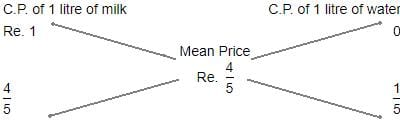Ratio of milk to water = 4/5 : 1/5
=> 4:5
Hence, percentage of water in the mixture = 1/5 x 100%
= 20%

Test: Alligation or Mixture- 2 - Question 9

In what ratio must water be mixed with milk costing Rs.12 per litre in order to get a mixture worth of Rs.8 per litre?

Detailed Solution for Test: Alligation or Mixture- 2 - Question 9

Let's assume cost of water is 0,
And the amount of water in mixture be x litres, and amount of milk in mixture be y litres,
So as per question the mixture would be sold a 8 Rs/litre
=> Cost of water per litre times amount of water in mixture of 1 litre + Cost of milk per litre times amount of milk in mixture of 1 litre must be equal to required cost of 1 litre mixture times 1 litre(total mixture quantity)
Mathematically, 0*x + 12*y = 8*1
=> 12 y = 8
=> y=2/3 litre
Now since total mixture is 1 litre,
So x = 1-y
x = 1-(2/3) = 1/3 litre
So ratio of water to milk in mixture, to get cost of required mixture to 1 litre = x/y = (1/3)/(2/3)
=> Ratio of water to milk in mixture is 1:2

Test: Alligation or Mixture- 2 - Question 10

In what ratio must water be mixed with milk to gain 16 2/3 % on selling the mixture at cost price?

Detailed Solution for Test: Alligation or Mixture- 2 - Question 10

Let C.P. of 1 litre milk be Rs. 1
S.P. of 1 litre of mixture = Rs. 1, Gain =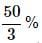∴ C.P. of 1 litre of mixture =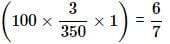BY the rule of alligation, we have: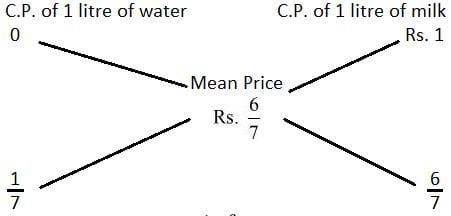∴ Ration of water and milk =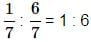## CSAT Preparation for UPSC CSE

72 videos|64 docs|92 tests
 Use Code STAYHOME200 and get INR 200 additional OFF Use Coupon Code
Information about Test: Alligation or Mixture- 2 Page
In this test you can find the Exam questions for Test: Alligation or Mixture- 2 solved & explained in the simplest way possible. Besides giving Questions and answers for Test: Alligation or Mixture- 2, EduRev gives you an ample number of Online tests for practice

## CSAT Preparation for UPSC CSE

72 videos|64 docs|92 tests